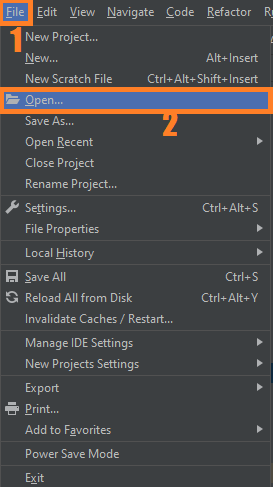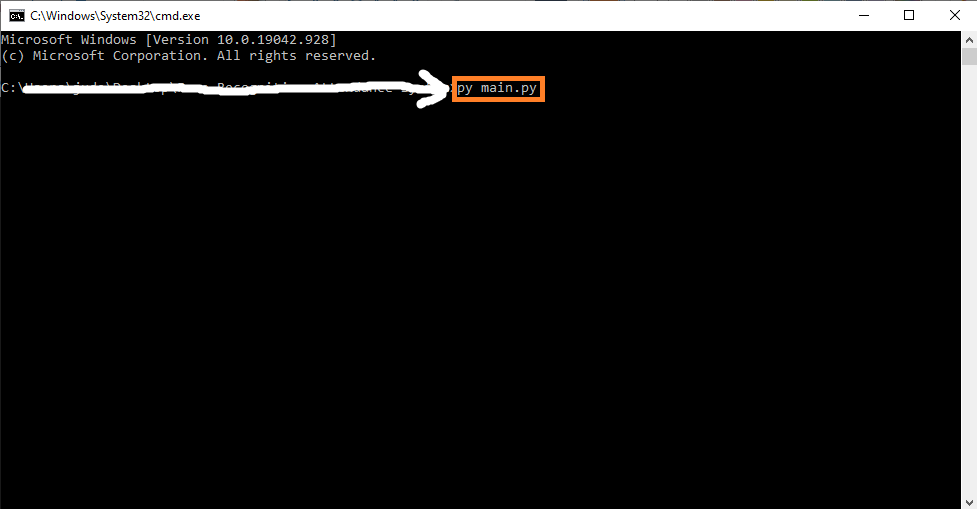# Cartoonify an Image OpenCV Python With Source Code

## Cartoonify an Image OpenCV Python With Source Code

The Cartoonify an Image OpenCV Python was developed using Python OpenCV, At the end of this article, we aim to transform images into its cartoon. Yes, we will CARTOONIFY the images. Thus, we will build a python application that will transform an image into its cartoon using OpenCV.

## What is OpenCV?

Python is the pool of libraries. It has numerous libraries for real-world applications. One such library is OpenCV. OpenCV is a cross-platform library used for Computer Vision. It includes applications like video and image capturing and processing. It is majorly used in image transformation, object detection, face recognition, and many other stunning applications.

To convert an image to a cartoon, multiple transformations are done. Firstly, an image is converted to a Grayscale image. Yes, similar to the old day’s pictures.! Then, the Grayscale image is smoothened, and we try to extract the edges in the image. Finally, we form a color image and mask it with edges. This creates a beautiful cartoon image with edges and lightened color of the original image.

By the way, if you are new to python programming and you don’t know what would be the Python IDE to use, I have here a list of the Best Python IDE for Windows, Linux, Mac OS that will suit you. I also have here How to Download and Install the Latest Version of Python on Windows.

To start executing Cartoonify an Image OpenCV Python With Source Code, make sure that you have installed Python 3.9 and PyCharm on your computer.

## Cartoonify an Image OpenCV Python With Source Code : Steps on how to run the project

Time needed: 5 minutes

These are the steps on how to run Cartoonify an Image OpenCV Python With Source Code

First, download the given source code below and unzip the source code.• Step 2: Import the project to your PyCharm IDE.• Step 3: Run the project.

last, run the project with the command “py main.py”## Installed Libraries

```import cv2 #for image processing
import easygui #to open the filebox
import numpy as np #to store image
import imageio #to read image stored at particular path

import sys
import matplotlib.pyplot as plt
import os
import tkinter as tk
from tkinter import filedialog
from tkinter import *
from PIL import ImageTk, Image```

## Complete Source Code

```import cv2 #for image processing
import easygui #to open the filebox
import numpy as np #to store image
import imageio #to read image stored at particular path

import sys
import matplotlib.pyplot as plt
import os
import tkinter as tk
from tkinter import filedialog
from tkinter import *
from PIL import ImageTk, Image

top=tk.Tk()
top.geometry('400x400')
top.configure(background='white')
label=Label(top,background='#CDCDCD', font=('calibri',20,'bold'))

ImagePath=easygui.fileopenbox()
cartoonify(ImagePath)

def cartoonify(ImagePath):
originalmage = cv2.cvtColor(originalmage, cv2.COLOR_BGR2RGB)
#print(image)  # image is stored in form of numbers

# confirm that image is chosen
if originalmage is None:
print("Can not find any image. Choose appropriate file")
sys.exit()

ReSized1 = cv2.resize(originalmage, (960, 540))
#plt.imshow(ReSized1, cmap='gray')

#converting an image to grayscale
grayScaleImage= cv2.cvtColor(originalmage, cv2.COLOR_BGR2GRAY)
ReSized2 = cv2.resize(grayScaleImage, (960, 540))
#plt.imshow(ReSized2, cmap='gray')

#applying median blur to smoothen an image
smoothGrayScale = cv2.medianBlur(grayScaleImage, 5)
ReSized3 = cv2.resize(smoothGrayScale, (960, 540))
#plt.imshow(ReSized3, cmap='gray')

#retrieving the edges for cartoon effect
#by using thresholding technique
cv2.THRESH_BINARY, 9, 9)

ReSized4 = cv2.resize(getEdge, (960, 540))
#plt.imshow(ReSized4, cmap='gray')

#applying bilateral filter to remove noise
#and keep edge sharp as required
colorImage = cv2.bilateralFilter(originalmage, 9, 300, 300)
ReSized5 = cv2.resize(colorImage, (960, 540))
#plt.imshow(ReSized5, cmap='gray')

#masking edged image with our "BEAUTIFY" image

ReSized6 = cv2.resize(cartoonImage, (960, 540))
#plt.imshow(ReSized6, cmap='gray')

# Plotting the whole transition
images=[ReSized1, ReSized2, ReSized3, ReSized4, ReSized5, ReSized6]

fig, axes = plt.subplots(3,2, figsize=(8,8), subplot_kw={'xticks':[], 'yticks':[]}, gridspec_kw=dict(hspace=0.1, wspace=0.1))
for i, ax in enumerate(axes.flat):
ax.imshow(images[i], cmap='gray')

save1.configure(background='#364156', foreground='white',font=('calibri',10,'bold'))

plt.show()

def save(ReSized6, ImagePath):
#saving an image using imwrite()
newName="cartoonified_Image"
path1 = os.path.dirname(ImagePath)
extension=os.path.splitext(ImagePath)
path = os.path.join(path1, newName+extension)
cv2.imwrite(path, cv2.cvtColor(ReSized6, cv2.COLOR_RGB2BGR))
I= "Image saved by name " + newName +" at "+ path
tk.messagebox.showinfo(title=None, message=I)

top.mainloop()

```